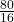## Rectangle HYGT has a perimeter of 170 inches. If the length is 10x – 7 and the width is 6x + 12, how wide is the rectangle?

Question

Rectangle HYGT has a perimeter of 170 inches. If the length is 10x – 7
and the width is 6x + 12, how wide is the rectangle?

in progress 0
3 weeks 2021-08-23T23:26:17+00:00 1 Answers 1 views 0

The wide of the rectangle = 42 inches

The  length of the rectangle = 43 inches

Step-by-step explanation:

Step(i):-

Given that the length of the rectangle = 10x-7

Given that the width of the rectangle = 6x +12

The perimeter of the rectangle = 2(length + width)

Given that the perimeter of the rectangle = 170

Step(ii):-

2(length + width) = 170

length + width = 85

10x-7 +6x +12 =85

16x +5 = 85

16x = 85-5 = 80

x =x = 5